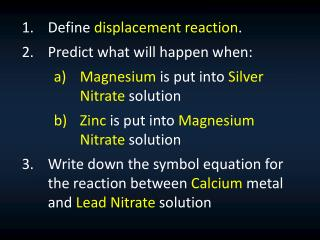DownloadDownload PresentationDefine displacement reaction . Predict what will happen when:

# Define displacement reaction . Predict what will happen when:

Download Presentation## Define displacement reaction . Predict what will happen when:

- - - - - - - - - - - - - - - - - - - - - - - - - - - E N D - - - - - - - - - - - - - - - - - - - - - - - - - - -
##### Presentation Transcript

1. Define displacement reaction. • Predict what will happen when: • Magnesium is put into Silver Nitrate solution • Zinc is put into Magnesium Nitrate solution • Write down the symbol equation for the reaction between Calcium metal and Lead Nitrate solution

2. When two solutions, Barium Nitrate and Sodium Hydroxide are mixed, the solution turns cloudy/milky – which indicates that precipitate has been formed. Write down the word equation for this reaction Write down the symbol equation for this reaction

3. Displacement Reaction It is a type of reaction which an element (or ion) replaces another element (or ion) in a compound. A + BC → B + AC Notice how ‘B’ has been replaced by ‘A’

4. Example ONE: Silver Nitrate & Copper When a piece of copper reacts with silver nitrate solution, the copper replaces the silver, producing copper nitrate and silver. Word equation: Symbol equation:

5. Precipitation Reaction (a.k.a. double exchange reaction) AB + CD → AD + CB BaNO3 + NaOH → Ba(OH)2 + NaNO3 Displacement Reaction (a.k.a. single exchange reaction) A + BC → B + AC Cu + AgNO3 → Ag + Cu(NO3)2

6. Example TWO: Copper Sulfate & Magnesium When a piece of magnesium is dropped into copper sulfatesolution, the magnesium replaces the copper, producing magnesium sulfateand copper. Word equation: Symbol equation:

7. Practical: Displacement Reaction between Magnesium and Copper Sulfate • Method: Drop a piece of magnesium ribbon into about 50 ml of copper sulfate solution in a beaker. • Result: • Word Equation: • Symbol Equation:

8. Displacement Reaction It is a type of reaction which an element (or ion) replaces another element (or ion) in a compound. A + BC → B + AC Notice how ‘B’ has been replaced by ‘A’

9. Displacement Reaction It is a type of reaction which an element (or ion) replaces another element (or ion) in a compound. WHY?

10. Bob = Copper Lara= Sulfate Nick= Magnesium • Laraditches Bob because she finds Nick more attractive. (i.e. Nick ‘replaces’ Bob) • In chemistry, “Attractive” means “Reactive”.

11. Reactivity Series of Metals Can you explain why magnesium replaces copper in copper sulfate solution?

12. What would happen…? Zinc + Copper sulfate → ??? Iron + copper sulfate → ??? Silver + copper sulfate → ??? Gold + + copper sulfate → ???

13. Practical: Displacement Reaction between Zinc / Iron and Copper Sulfate Add some zinc granules to about 50 ml of copper sulfate solution in a test tube. Write down the result, word equation and balanced symbol equation Repeat the whole experiment, using iron fillingsinstead of zinc granules

14. Practical: Reactivity Series Method: In five test tubes, put about 5 ml of copper sulfatesoultion. Put a piece of copper, zinc, tin, lead and magnesium into the test tubes. Prediction: Which will react and which will NOT react? Why and why not?

15. Write down the result • Write down the word equationsAND the balanced symbol equations for the reactions that did take place.

16. Practical: Reactivity Series 2 Task - create your own reactivity series